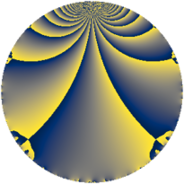# Properties

 Label 7800.2.a.bjLevel $7800$ Weight $2$ Character orbit 7800.a Self dual yes Analytic conductor $62.283$ Analytic rank $0$ Dimension $3$ CM no Inner twists $1$

# Related objects

## Newspace parameters

 Level: $$N$$ $$=$$ $$7800 = 2^{3} \cdot 3 \cdot 5^{2} \cdot 13$$ Weight: $$k$$ $$=$$ $$2$$ Character orbit: $$[\chi]$$ $$=$$ 7800.a (trivial)

## Newform invariants

 Self dual: yes Analytic conductor: $$62.2833135766$$ Analytic rank: $$0$$ Dimension: $$3$$ Coefficient field: 3.3.568.1 Defining polynomial: $$x^{3} - x^{2} - 6 x - 2$$ Coefficient ring: $$\Z[a_1, \ldots, a_{11}]$$ Coefficient ring index: $$2$$ Twist minimal: no (minimal twist has level 1560) Fricke sign: $$-1$$ Sato-Tate group: $\mathrm{SU}(2)$

## $q$-expansion

Coefficients of the $$q$$-expansion are expressed in terms of a basis $$1,\beta_1,\beta_2$$ for the coefficient ring described below. We also show the integral $$q$$-expansion of the trace form.

 $$f(q)$$ $$=$$ $$q - q^{3} + q^{9} +O(q^{10})$$ $$q - q^{3} + q^{9} + ( -2 - \beta_{1} ) q^{11} - q^{13} + ( 1 - \beta_{1} - \beta_{2} ) q^{17} + ( -1 - \beta_{1} - \beta_{2} ) q^{19} + ( 3 - \beta_{1} - \beta_{2} ) q^{23} - q^{27} + ( -4 - 2 \beta_{2} ) q^{29} + ( 4 + 2 \beta_{1} ) q^{31} + ( 2 + \beta_{1} ) q^{33} + ( -2 - 2 \beta_{1} ) q^{37} + q^{39} + ( -3 - 2 \beta_{1} + \beta_{2} ) q^{41} + ( -1 + \beta_{1} + \beta_{2} ) q^{43} + ( 5 - 2 \beta_{1} - \beta_{2} ) q^{47} -7 q^{49} + ( -1 + \beta_{1} + \beta_{2} ) q^{51} + ( 4 + 2 \beta_{2} ) q^{53} + ( 1 + \beta_{1} + \beta_{2} ) q^{57} + ( -2 + 3 \beta_{1} ) q^{59} + ( 1 - \beta_{1} + \beta_{2} ) q^{61} + ( 2 - 2 \beta_{1} + 2 \beta_{2} ) q^{67} + ( -3 + \beta_{1} + \beta_{2} ) q^{69} + ( 3 + \beta_{2} ) q^{71} + ( 2 + 4 \beta_{1} ) q^{73} + ( 7 + \beta_{1} + \beta_{2} ) q^{79} + q^{81} + ( 1 + 2 \beta_{1} - \beta_{2} ) q^{83} + ( 4 + 2 \beta_{2} ) q^{87} + ( -7 - 2 \beta_{1} + \beta_{2} ) q^{89} + ( -4 - 2 \beta_{1} ) q^{93} + 6 q^{97} + ( -2 - \beta_{1} ) q^{99} +O(q^{100})$$ $$\operatorname{Tr}(f)(q)$$ $$=$$ $$3q - 3q^{3} + 3q^{9} + O(q^{10})$$ $$3q - 3q^{3} + 3q^{9} - 6q^{11} - 3q^{13} + 4q^{17} - 2q^{19} + 10q^{23} - 3q^{27} - 10q^{29} + 12q^{31} + 6q^{33} - 6q^{37} + 3q^{39} - 10q^{41} - 4q^{43} + 16q^{47} - 21q^{49} - 4q^{51} + 10q^{53} + 2q^{57} - 6q^{59} + 2q^{61} + 4q^{67} - 10q^{69} + 8q^{71} + 6q^{73} + 20q^{79} + 3q^{81} + 4q^{83} + 10q^{87} - 22q^{89} - 12q^{93} + 18q^{97} - 6q^{99} + O(q^{100})$$

Basis of coefficient ring in terms of a root $$\nu$$ of $$x^{3} - x^{2} - 6 x - 2$$:

 $$\beta_{0}$$ $$=$$ $$1$$ $$\beta_{1}$$ $$=$$ $$\nu^{2} - \nu - 4$$ $$\beta_{2}$$ $$=$$ $$-\nu^{2} + 3 \nu + 3$$
 $$1$$ $$=$$ $$\beta_0$$ $$\nu$$ $$=$$ $$($$$$\beta_{2} + \beta_{1} + 1$$$$)/2$$ $$\nu^{2}$$ $$=$$ $$($$$$\beta_{2} + 3 \beta_{1} + 9$$$$)/2$$

## Embeddings

For each embedding $$\iota_m$$ of the coefficient field, the values $$\iota_m(a_n)$$ are shown below.

For more information on an embedded modular form you can click on its label.

Label $$\iota_m(\nu)$$ $$a_{2}$$ $$a_{3}$$ $$a_{4}$$ $$a_{5}$$ $$a_{6}$$ $$a_{7}$$ $$a_{8}$$ $$a_{9}$$ $$a_{10}$$
1.1
 3.12489 −1.76156 −0.363328
0 −1.00000 0 0 0 0 0 1.00000 0
1.2 0 −1.00000 0 0 0 0 0 1.00000 0
1.3 0 −1.00000 0 0 0 0 0 1.00000 0
 $$n$$: e.g. 2-40 or 990-1000 Significant digits: Format: Complex embeddings Normalized embeddings Satake parameters Satake angles

## Atkin-Lehner signs

$$p$$ Sign
$$2$$ $$1$$
$$3$$ $$1$$
$$5$$ $$-1$$
$$13$$ $$1$$

## Inner twists

This newform does not admit any (nontrivial) inner twists.

## Twists

By twisting character orbit
Char Parity Ord Mult Type Twist Min Dim
1.a even 1 1 trivial 7800.2.a.bj 3
5.b even 2 1 7800.2.a.bp 3
5.c odd 4 2 1560.2.l.c 6
15.e even 4 2 4680.2.l.e 6
20.e even 4 2 3120.2.l.m 6

By twisted newform orbit
Twist Min Dim Char Parity Ord Mult Type
1560.2.l.c 6 5.c odd 4 2
3120.2.l.m 6 20.e even 4 2
4680.2.l.e 6 15.e even 4 2
7800.2.a.bj 3 1.a even 1 1 trivial
7800.2.a.bp 3 5.b even 2 1

## Hecke kernels

This newform subspace can be constructed as the intersection of the kernels of the following linear operators acting on $$S_{2}^{\mathrm{new}}(\Gamma_0(7800))$$:

 $$T_{7}$$ $$T_{11}^{3} + 6 T_{11}^{2} + 2 T_{11} - 20$$ $$T_{17}^{3} - 4 T_{17}^{2} - 20 T_{17} + 64$$ $$T_{19}^{3} + 2 T_{19}^{2} - 24 T_{19} + 16$$

## Hecke characteristic polynomials

$p$ $F_p(T)$
$2$ $$T^{3}$$
$3$ $$( 1 + T )^{3}$$
$5$ $$T^{3}$$
$7$ $$T^{3}$$
$11$ $$-20 + 2 T + 6 T^{2} + T^{3}$$
$13$ $$( 1 + T )^{3}$$
$17$ $$64 - 20 T - 4 T^{2} + T^{3}$$
$19$ $$16 - 24 T + 2 T^{2} + T^{3}$$
$23$ $$80 + 8 T - 10 T^{2} + T^{3}$$
$29$ $$-472 - 44 T + 10 T^{2} + T^{3}$$
$31$ $$160 + 8 T - 12 T^{2} + T^{3}$$
$37$ $$-136 - 28 T + 6 T^{2} + T^{3}$$
$41$ $$-332 - 34 T + 10 T^{2} + T^{3}$$
$43$ $$-64 - 20 T + 4 T^{2} + T^{3}$$
$47$ $$256 + 34 T - 16 T^{2} + T^{3}$$
$53$ $$472 - 44 T - 10 T^{2} + T^{3}$$
$59$ $$44 - 78 T + 6 T^{2} + T^{3}$$
$61$ $$32 - 32 T - 2 T^{2} + T^{3}$$
$67$ $$256 - 128 T - 4 T^{2} + T^{3}$$
$71$ $$64 + 2 T - 8 T^{2} + T^{3}$$
$73$ $$824 - 148 T - 6 T^{2} + T^{3}$$
$79$ $$-160 + 108 T - 20 T^{2} + T^{3}$$
$83$ $$232 - 62 T - 4 T^{2} + T^{3}$$
$89$ $$-244 + 94 T + 22 T^{2} + T^{3}$$
$97$ $$( -6 + T )^{3}$$